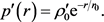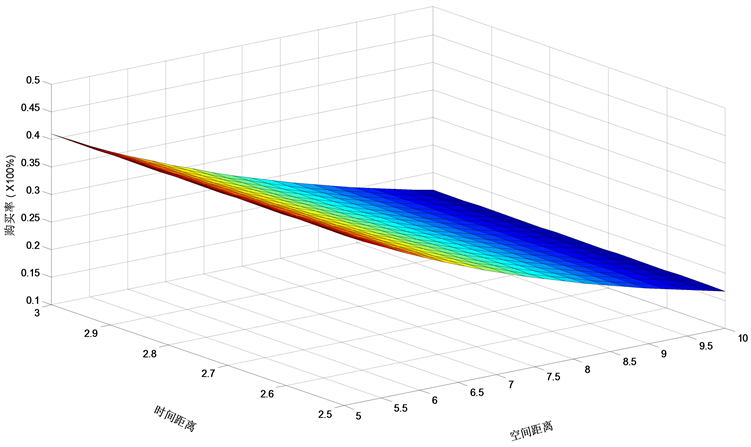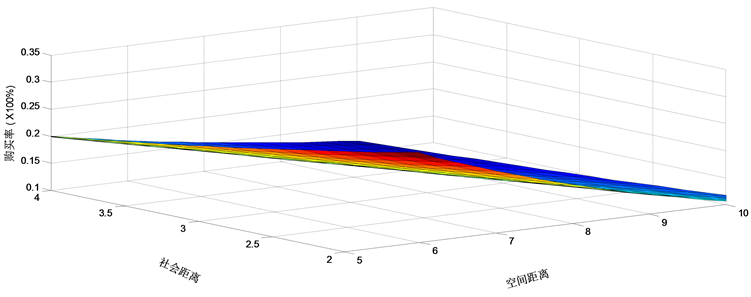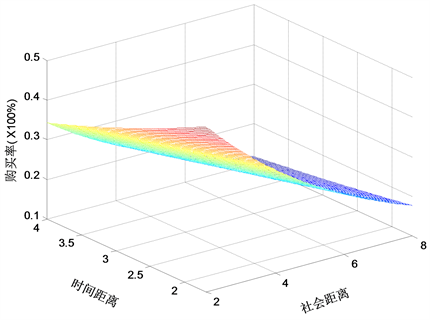# 在线消费者心理距离与决策行为的关系模型Modelling a New Relation between the Consumption Decision and Psychological Distance of Online Consumers

DOI: 10.12677/MSE.2018.74027, PDF, HTML, XML, 下载: 729  浏览: 1,818  国家自然科学基金支持

Abstract: In the age of e-commence, numerous characteristics of psychic distance are distinct from that of traditional markets. To better understand these differences, in this present paper, we established a new relationship between consumption decision and psychological distance of online consumers by making use of the Maximum Entropy Principle. According to the classification of the commodities online, we focus on printed books and digital products. Data were collected from the China-based B2C e-commerce platform, Jingdong and Alibaba’s Taobao Mall, by convenience sampling. Data collection yielded 302 valid questionnaires. The results showed that the psychological distance has a negative influence on the consumption decision of online consumers; the three dimensions of psychological distance can significantly influence the online consumer buying behavior, and the impact from high to low in order is: time distance, social distance and spatial distance. By this technique, an in-depth methodology for establishing the interaction between the consumption decision and psychological distance is presented for better marketing strategy of e-business.

1. 引言

2. 熵与最大熵原理

$S=k\mathrm{log}W,$ (2.1)

$H=-K\underset{i}{\sum }{P}_{i}\mathrm{ln}{P}_{i}\text{ }\text{ }.$ (2.2)

1) 离散型

$H=-\underset{i=1}{\overset{n}{\sum }}{p}_{i}\mathrm{ln}{p}_{i}.$ (2.3)

$\underset{i=1}{\overset{n}{\sum }}{p}_{i}=1,\text{\hspace{0.17em}}{p}_{i}\ge 0,i=1,2,\cdots ,n\text{\hspace{0.17em}}.$ (2.4)

$\begin{array}{l}\underset{i=1}{\overset{n}{\sum }}{p}_{i}=1,{p}_{i}\ge 0,i=1,2,\cdots ,n\text{\hspace{0.17em}};\\ \underset{i=1}{\overset{n}{\sum }}{p}_{i}{x}_{i}=E.\end{array}$ (2.5)

2) 连续型

$H=-\int f\left(x\right)\mathrm{ln}f\left(x\right)\text{d}\left(x\right).$ (2.6)

$H=\int f\left(x\right)\text{d}\left(x\right)=1,\text{\hspace{0.17em}}\left(f\left(x\right)\ge 0\right).$ (2.7)

$\begin{array}{l}\int f\left(x\right)\text{d}x=1\text{ };\\ \int xf\left(x\right)\text{d}x=E.\end{array}$ (2.8)

3. 心理距离与决策行为关系模型构建

$W=\left(\begin{array}{c}P\\ {p}_{0},{p}_{1},\cdots ,{p}_{n}\end{array}\right)=P!/\underset{i=0}{\overset{n}{\prod }}{p}_{i}!.$ (3.1)

$H=\mathrm{ln}W=\mathrm{ln}P!\text{\hspace{0.17em}}-\underset{i=0}{\overset{n}{\sum }}\mathrm{ln}{p}_{i}!.$ (3.2)

$\underset{i=0}{\overset{n}{\sum }}{p}_{i}=P;$ (3.3)

$\underset{i}{\sum }2\text{π}i{p}_{i}={P}_{0},$ (3.4)

$L\left({p}_{i}\right)=\mathrm{ln}P!\text{\hspace{0.17em}}-\underset{i=0}{\overset{n}{\sum }}\mathrm{ln}{p}_{i}!+{\lambda }_{1}\left(P-\underset{i=0}{\overset{n}{\sum }}{p}_{i}\right)+{\lambda }_{2}\left({P}_{0}-\underset{i=0}{\overset{n}{\sum }}2\text{π}i{p}_{i}\right).$ (3.5)

${p}_{i}={\text{e}}^{-{\lambda }_{1}}\cdot {\text{e}}^{-2\text{π}i{\lambda }_{2}}.$ (3.6)

${\int }_{0}^{R}2\text{π}r{\text{e}}^{-{\lambda }_{1}}\cdot {\text{e}}^{-2\text{π}r{\lambda }_{2}}\text{d}r={P}_{0}.$ (3.7)

$p\left(r\right)=2\text{π}{\lambda }_{2}^{2}{P}_{0}{\text{e}}^{-2\text{π}r{\lambda }_{2}}.$ (3.8)

${\rho }_{0}=2\text{π}{\lambda }_{2}^{2}{P}_{0}$ (3.9)

${\rho }_{0}=\frac{{P}_{0}}{2\text{π}{r}_{0}^{2}}.$ (3.10)

$p\left(r\right)={\rho }_{0}{\text{e}}^{-r/{r}_{0}}.$ (3.11)

$PD=\alpha TD+\beta {S}_{social}D+\gamma {S}_{spatial}D,$ (3.12)

$p\left(x,y,z\right)={\rho }_{0}{\text{e}}^{-\left(\alpha x+\beta \delta y+\gamma z\right)/{r}_{0}}.$ (3.13)(3.14)

${p}^{\prime }\left(x,y,z\right)={{\rho }^{\prime }}_{0}{\text{e}}^{-\left({\alpha }^{\prime }x+{\beta }^{\prime }y+{\gamma }^{\prime }z\right)/{r}_{0}}.$ (3.15)

$\upsilon =\frac{{p}^{\prime }\left(x,y,z\right)}{p\left(x,y,z\right)}={{\rho }^{\prime }}_{0}/\rho \cdot {\text{e}}^{\left(\left(\alpha -{\alpha }^{\prime }\right)x+\left(\beta -{\beta }^{\prime }\right)y+\left(\gamma -{\gamma }^{\prime }\right)z\right)/{r}_{0}}$ (3.16)

4. 模型验证Table 1. Measure of time distanceTable 2. Measure of social distanceTable 3. Measure of spatial distanceTable 4. Results of fitting curve in (3.18)Figure 1. A decreasing surface meaning the relation between purchase rate and time distance and spatial distanceFigure 2. A decreasing surface meaning the relation between purchase rate and social distance and spatial distanceFigure 3. A decreasing surface meaning the relation between purchase rate and time distance and social distance

5. 结论

  Frances, C. (2001) The Death of Distance 2.0: How the Communications Revolution Will Change Our Lives. Texere, New York.  Hipp, J.R. (2010) Micro-Structure in Micro-Neighborhoods: A New Social Distance Measure, and Its Effect on Individual and Aggregated Perceptions of Crime and Disorder. Social Networks, 32, 148-159. https://doi.org/10.1016/j.socnet.2009.11.001  Griswold, W. and Wright, N. (2004) Cowbirds, Locals, and the Dynamic Endurance of Regionalism. American Journal of Sociology, 109, 1411-1451. https://doi.org/10.1086/381773  Edwards, S.M., Lee, J.K. and La Ferle, C. (2009) Does Place Matter When Shopping Online? Perceptions of Similarity and Familiarity as Indicators of Psychological Distance. Journal of Interactive Advertising, 10, 35-50. https://doi.org/10.1080/15252019.2009.10722161  Benedicktus, R.L. (2008) Psychological Distance Perceptions and Trust Beliefs for Internet-Only and Hybrid Retailers: Implications for Marketers. The Florida State University, Tallahassee.  Kim, K., Zhang, M. and Li, X. (2008) Effects of Temporal and Social Distance on Consumer Evaluations. Journal of Consumer Research, 35, 706-713. https://doi.org/10.1086/592131  Bornemann, T. and Homburg, C. (2011) Psychological Distance and the Dual Role of Price. Journal of Consumer Research, 38, 490-504. https://doi.org/10.1086/659874  Huang, N., et al. (2016) Effects of Multiple Psychological Distances on Construal and Consumer Evaluation: A Field Study of Online Reviews. Journal of Consumer Psychology, 26, 474-482. https://doi.org/10.1016/j.jcps.2016.03.001  Yamin, M. and Sinkovics, R.R. (2006) Online Internationalisation, Psychic Distance Reduction and the Virtuality Trap. International Business Review, 15, 339-360. https://doi.org/10.1016/j.ibusrev.2006.03.002  Bar-Anan, Y., Liberman, N. and Trope, Y. (2006) The Association between Psychological Distance and Construal Level: Evidence from an Implicit Association Test. Journal of Experimental Psychology: General, 135, 609. https://doi.org/10.1037/0096-3445.135.4.609  Wiebe, J., Basil, D.Z. and Runté, M. (2017) Psychological Distance and Perceived Consumer Effectiveness in a Cause-Related Marketing Context. International Review on Public and Nonprofit Marketing, 14, 197-215. https://doi.org/10.1007/s12208-016-0170-y  Liu, Y. and Xu, J. (2015) The Effect of Temporal Distance and Social Distance on the Choice of Consumers’ Preferences. Modern Economy, 6, 275. https://doi.org/10.4236/me.2015.62024  Lim, S., et al. (2012) Getting Closer and Experiencing Together: Antecedents and Consequences of Psychological Distance in Social Media-Enhanced Real-Time Streaming Video. Computers in Human Behavior, 28, 1365-1378. https://doi.org/10.1016/j.chb.2012.02.022  黄鹤婷, 赵冬梅. 在线消费者的心理距离及其测度方法研究——基于解释水平理论的视角[J]. 经济研究参考, 2013(14): 42-48.  Wang, L., Zhao, D. and Huang, H. (2013) An Empirical Analysis of the Online Consumer’s Psychological Distance. International Conference on Management Science and Engineering, Harbin, 17-19 July 2013, 78-83.  Lalazissis, G.A., Massen, S.E., Panos, C.P. and Dimitrova, S.S. (1998) Information Entropy as a Measure of the Quality of a Nuclear Density Distribution. International Journal of Modern Physics E, 7, 485-494. https://doi.org/10.1142/S0218301398000257  Zhao, M. and Xie, J. (2011) Effects of Social and Temporal Distance on Consumers’ Responses to Peer Recommendations. Journal of Marketing Research, 48, 486-496. https://doi.org/10.1509/jmkr.48.3.486  Barron, A.R. (1986) Entropy and the Central Limit Theorem. The Annals of Proba-bility, 14, 336-342. https://doi.org/10.1214/aop/1176992632  Georgii, H.-O. (2003) Probabilistic Aspects of Entropy. In: Greven, A., Keller, G. and Warnecke, G., Eds., Entropy, Princeton University Press, Princeton, 37-54.  Lewis, J.T., Pfister, C.-E. and Sullivan, W.G. (1995) Entropy, Concentration of Probability and Conditional Limit Theorems. Markov Processes and Related Fields, 1, 319-386.  Maes, C., Redig, F. and Van Moffaert, A. (2000) On the Definition of Entropy Production, via Examples. Journal of Mathematical Physics, 41, 1528-1554. https://doi.org/10.1063/1.533195  Csiszar, I. and Körner, J. (2011) Information Theory: Coding Theorems for Discrete Memoryless Systems. Cambridge University Press, Cambridge. https://doi.org/10.1017/CBO9780511921889  Shannon, C.E. (1948) A Mathematical Theory of Communication. Bell System Technical Journal, 27, 379. https://doi.org/10.1002/j.1538-7305.1948.tb01338.x  Bialynicki-Birula, I. and Mycielski, J. (1975) Uncertainty Relations for Information Entropy in Wave Mechanics. Communications in Mathematical Physics, 44, 129-132. https://doi.org/10.1007/BF01608825  Brooks, D.R., Wiley, E.O. and Brooks, D.R. (1988) Evolution as Entropy. University of Chicago Press, Chicago.  Ulanowicz, R.E. and Hannon, B.M. (1987) Life and the Production of Entropy. Proceedings of the Royal Society of London B, 232, 181-192. https://doi.org/10.1098/rspb.1987.0067  Jaynes, E.T. (1957) Information Theory and Statistical Mechanics. Physical Review, 106, 620. https://doi.org/10.1103/PhysRev.106.620  Kullback, S. (1959) Information Theory and Statistics. Wiley, New York.  Kapur, J.N. and Kesavan, H.K. (1992) Entropy Optimization Principles and Their Applications. Entropy and Energy Dissipation in Water Resources. Springer, Dordrecht, 3-20. https://doi.org/10.1007/978-94-011-2430-0_1  Annila, A. and Salthe, S. (2009) Economies Evolve by Energy Dispersal. Entropy, 11, 606-633. https://doi.org/10.3390/e11040606  Funkhouser, S. (2012) The Entropy of a Discrete Real Variable. Entropy, 14, 1522-1538. https://doi.org/10.3390/e14081522  Clark, C. (1951) Urban Population Densities. Journal of the Royal Statistical Society. Series A (General), 114, 490-496. https://doi.org/10.2307/2981088  Wilson, A.G. (2011) Entropy in Urban and Regional Modelling. Vol. 1, Routledge, Ab-ingdon-on-Thames.